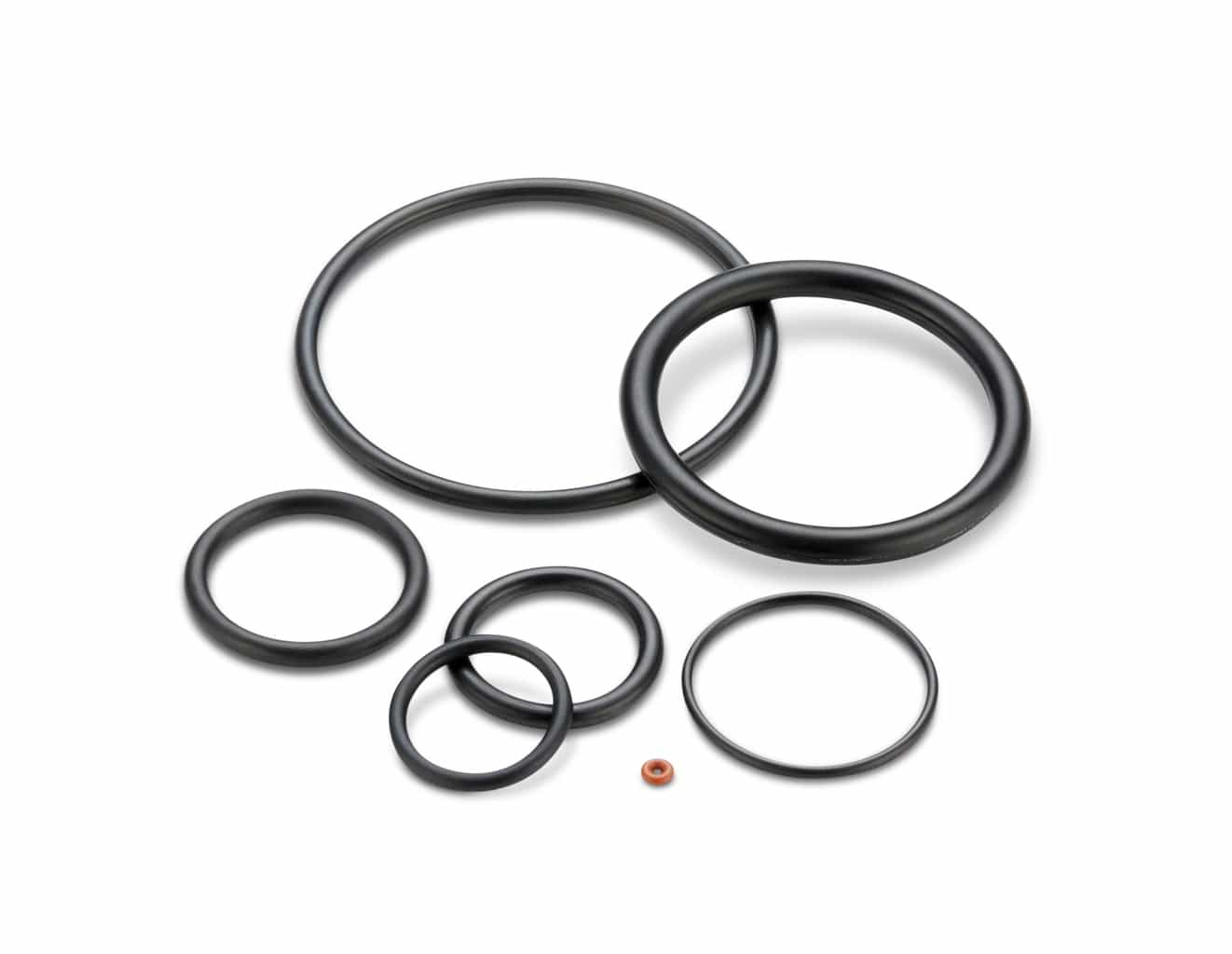# Piston Seal Application Example

## Application Example: Piston Quad-Ring® Brand Seal

#### Application description: Hydraulic Cylinder, U. S. Customary Units (inches)

• 5″ dynamic stroke
• Piston diameter: 2.992″ ±0.002
• Bore diameter: 3.000″ ±0.002
• 200 psi maximum pressure
• 0.103″ cross-section Quad-Ring® Brand seal

1. Calculate the Seal Groove Diameter:
Groove Diameter
= Maximum Bore Diameter – (2 x Dynamic Gland Depth)
= 3.002 – ( 2 x 0.094)
= 2.814 -0.000/+ 0.002
(Recall the gland depth values in the chart are given as radial values)

2. From the chart, the groove width is 0.115 -0.000/+0.005

3. Calculate the Minimum Gland Cross-sectional Area:
Minimum Gland Volume
= ((Min Bore Dia. – Max Groove Dia./ 2) x Min Groove Width
= ((2.998 – 2.816 )/2) X 0.115
= 0.0105 in2

4. Calculate the Maximum Quad-Ring® Brand Seal Cross-sectional Area:
= (Max Quad-Ring® Brand Cross-section)2 X 0.8215
= (0.106)2 X 0.8215
= 0.0092 in2

5. Compare the Minimum Gland Volume to the Maximum Quad-Ring® Brand Volume
In this application the maximum seal volume is less than the minimum gland volume,
so the seal should function satisfactorily.

6. Calculate the Minimum and Maximum Seal Squeeze
a.
Max Seal Squeeze = 1 – (Min Gland Depth / Max Seal Cross-section)
Min Gland Depth = (Min Bore Dia. – Max Groove Dia.) / 2
= (2.998 – 2.816) / 2
= 0.091
Max Seal Squeeze = 1 – (0.091/0.106)
= 0.141
= 14.1%
b. Min Seal Squeeze = 1 – (Max Gland Depth / Min Seal Cross-section)
Max Gland Depth = (Max Bore Dia. – Min Groove Dia.) / 2
= (3.002 – 2.814) / 2
= 0.094
Min Seal Squeeze = 1 – (0.094/0.100)
= 0.06
= 6%
Therefore, sufficient squeeze should exist to seal this application.

7. Calculate the Maximum Clearance and evaluate possible extrusion problems
Max Radial Clearance = (Max Bore Dia. – Min Piston Dia.) / 2
= (3.002 – 2.990) /2
= 0.006
From the Clearance Chart, the recommended max clearance for a Quad-Ring® Brand with a hardness of 70 Shore A at 200 psi is 0.009. The seal should function properly.

8. Select the Seal Size
Refer to the Selection Guide and turn to the section which lists the seals having a 0.103 cross-section. Since in this application the sealing is occurring on the bore, use the Bore column to look up the seal size for a 3.000″ bore. The correct seal is a number 4 -149 (with the 4 prefix signifying a Quad-Ring® Brand seal). Note the seal inside diameter, which is 2.800 ±0.022. This will be used below.

9. Calculate the Installed Seal Stretch
Stretch % = ((Installed Seal ID – Original Seal Inside Diameter) /
Original Seal Diameter) x 100
= ((Groove Diameter – Original Seal Inside Diameter) /
Original Seal Diameter) x 100
= ((2.814 – 2.800) / 2.800) x 100
= (0.014 / 2.800) * 100 = 0.5 %
This stretch is low and will not cause significant cross-sectional reduction.